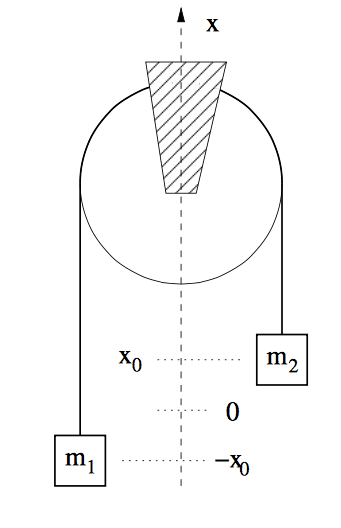# Pulleys & Masses: Instantaneous Acceleration

• struggles
In summary, the instantaneous acceleration of m2 in the case of a string of length l with mass per length λ ̸= 0 is given by a2 = (m1 −m2)+2x0λg/(M + m1 + m2), where M = λ l is the total mass of the string. This can be derived by doing a force balance on the string and setting it equal to the acceleration of the string as a whole. Equations T2 - m2g = m2a and T1 - m1g = -m1a can also be used in this case. Equations T1- m1g - (l/2 + x0)λg = m1

## Homework Statement

Consider now the case where the string of length l has mass per length λ ̸= 0. Show that the instantaneous acceleration of m2 is given by a2 = (m1 −m2)+2x0λg/(M + m1 + m2)
where M = λ l is the total mass of the string and find the tension in both ends of the string. (please see picture!)## Homework Equations

I have the solution and it starts off with three equations
T2 - m2g = m2a
T1 - m1g = -m1a
T1 + 2x0λg - T2 = Ma2

Although i completely understand where the first two come from I'm a little confused my the last one. Although i get that it is supposed to show the force on the string, I can't figure out how the left hand side is true. IF someone could please explain where this equation came from that would be much appreciated.

Also when i was attempting this problem before seeing the solution i came up with two equations:
T1- m1g - (l/2 + x0)λg = m1a and the equivalent for the second mass. Would these also be correct?

## The Attempt at a Solution

#### Attachments

struggles said:
T1 + 2x0λg - T2 = Ma2

Although i completely understand where the first two come from I'm a little confused my the last one. Although i get that it is supposed to show the force on the string, I can't figure out how the left hand side is true. IF someone could please explain where this equation came from that would be much appreciated.
The trailing sub-2 on the Ma appears to be a typo. There is only one acceleration, a.

Do a force balance on the string. We have downward force on the one end, T1. We have downward force on the other end, T2. Those two subtract as shown. We have downward force on the string where it hangs over the pulley on the one side down to the position of m2. And an identical force on the other side. Those two cancel. We have an extra length of 2x0 of string on the left hand side. The force from gravity on that segment that contributes a term. Sum those up and set it to the acceleration of the string as a whole and you get the equation as shown.

struggles said:
as attempting this problem before seeing the solution i came up with two equations:
T1- m1g - (l/2 + x0)λg = m1a and the equivalent for the second mass. Would these also be correct?
No. I suspect you are not being consistent in your definition of T1 to arrive at that. Also, the l/2 term is problematic however you define the tension, since some of the l/2+x0 is not vertical.

======================
JB444 has made my initial bit redundant, but
i came up with two equations:
T1- m1g - (l/2 + x0)λg = m1a and the equivalent for the second mass. Would these also be correct?
The (l/2 + x0)λg term represents the weight of half the string plus x0. I can't quite see that myself.
When x0=0, then there is L/2 on each side balancing each other - no net weight in either direction.
When the masses have moved by a finite x0, the string on one side has increased by x0, but the other side has also reduced by x0. The net force due to the weight of string is +x0 -(-x0), as the solution and JB said.
My other doubt about your equation (I'm not sure what your second equivalent one will be) is the directions of T1 and a. Until you either show the direction of a on your diagram, or give the second equation, I can't be sure whether you're wrong or have just changed the direction of a.

Thank you all for your help. I think i understand now :)